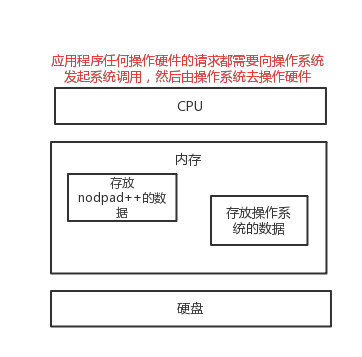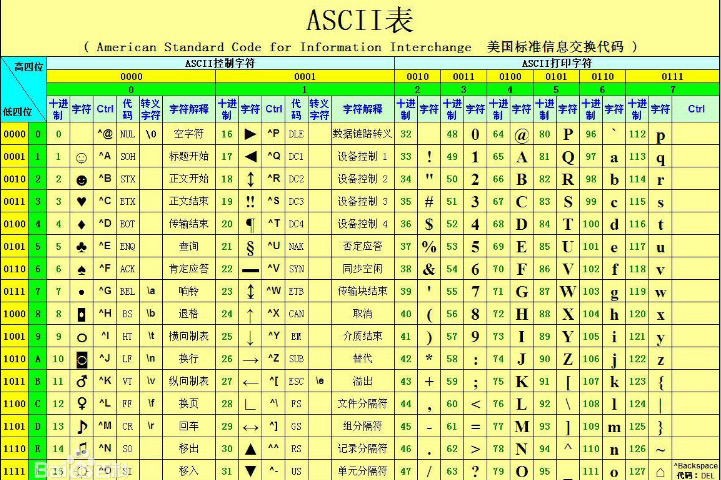# 1. is == id

### 1.3 id：测试对象内存地址

a = 'abc'

b = 'abc'

print(a == b) # True

print(a is b) # True

print(id(a)，id(b))

### 1.4 小数据池 int str

##### 1.4.1 int：-5 -- 256
a = 123 b = 123 c = 123

print)(id(a),id(b),id(c)) # id一样
##### 1.4.2 str：

1. 不能含有特殊字符

2. 单个因素*int不能超过21

# 2. 字符编码

### 2.1 了解字符编码的知识储备

##### 2.1.1 计算机基础知识1）打开编辑器就打开了启动了一个进程，是在内存中的，所以，用编辑器编写的内容也都是存放在内存中的，断电后数据丢失。

2）要想永久保存，需要点击保存按钮：编辑器把内存的数据刷到了硬盘上。

3）在我们编写一个py文件（没有执行），跟编写其他文件没有任何区别，都只是在编写一堆字符而已。

### 2.2 字符编码

##### 2.2.1 ASCII

ASCII（American Standard Code for Information Interchange，美国标准信息交换代码）是基于拉丁字母的一套电脑编码系统，主要用于显示现代英语和其他西欧语言，其最多只能用 8 位来表示（一个字节），即：2**8 = 256，所以，ASCII码最多只能表示 256 个符号。##### 2.2.2 Unicode

Unicode（统一码、万国码、单一码）是一种在计算机上使用的字符编码。Unicode 是为了解决传统的字符编码方案的局限而产生的，它为每种语言中的每个字符设定了统一并且唯一的二进制编码，规定虽有的字符和符号最少由 16 位来表示（2个字节），即：2 **16 = 65536，

##### 2.2.3 UTF-8

UTF-8，是对Unicode编码的压缩和优化，他不再使用最少使用2个字节，而是将所有的字符和符号进行分类：ascii码中的内容用1个字节保存、欧洲的字符用2个字节保存，东亚的字符用3个字节保存。目前公认最好的编码方式就是UTF-8。

##### 2.2.4 GBK

GBK是国标，只识别中文和英文，采用单双字节编码，英文使用单字节编码，完全兼容ASCII，中文部分采用双字节编码。

8bit  = 1bytes

1024bytes = 1kb

1024kb = 1mb

1024mb = 1GB

1024GB = 1TB

### 2.3 Python3下的编码转化

##### 2.3.1 python3环境下

1、不同编码之间的二进制是不能互相识别的；

2、python3，str内部编码方式（内存）为unicode。

3、对于文件的存贮和传输不能用unicode，bytes类型内部编码方式，为非unicode

##### 2.3.2 str与bytes的表现形式

# str表现形式，内部编码方式：unicode

s1 = 'hello'

# bytes表现形式，内部编码方式：非unicode（utf-8,gbk....）

s2 = b'hello'

# 对于英文

s1 = 'hello'

print(s1, type(s1)) # hello <class 'str'>

s2 = b'hello'

print(s2, type(s2)) # b'hello' <class 'bytes'>

# 对于中文

s = '你好'

print(s, type(s)) # 你好 <class 'str'>

print(s.encode('utf-8')) # b'\xe4\xbd\xa0\xe5\xa5\xbd'

# s1=b'\xe4\xbd\xa0\xe5\xa5\xbd'

print(s1, type(s1)) # b'\xe4\xbd\xa0\xe5\xa5\xbd' <class 'bytes'>

##### 2.3.4 编码转化：str 转化成 bytes
# str(英文)转化 bytes

s = 'hello'

s2 = s.encode('utf-8')

s3 = s.encode('gbk')

print(s2, type(s2)) # >>>b'hello' <class 'bytes'>

print(s3, type(s3)) # >>>b'hello' <class 'bytes'>

# str(中文)转化 bytes

s = '你好'

s2 = s.encode('utf-8') # 一个中文三个字节

s3 = s.encode('gbk') # 一个中文两个字节

print(s2, type(s2)) # b'\xe4\xbd\xa0\xe5\xa5\xbd' <class 'bytes'>

print(s3, type(s3)) # b'\xc4\xe3\xba\xc3' <class 'bytes'>

##### 2.3.5 解码：bytes 转化成str
s = '你好'

s1 = s.encode('utf-8') # 一个中文三个字节

s2 = s.encode('gbk') # 一个中文两个字节

s3 = s1.decode('utf-8')

s4 = s2.decode('gbk')

print(s3, type(s3)) # >>>你好 <class 'str'>

print(s4, type(s4)) # >>>你好 <class 'str'>

##### 2.3.6 编码方式补充：
unicode - --> bytes

encode()

bytes - --> unicode

decode()

# 将gbk的bytes类型转化为utf-8的bytes类型

s1 = b'\xd6\xd0\xb9\xfa'

s2 = s1.decode('gbk')

s3 = s2.encode('utf-8')

print(s2) # 中国

# 简化

s1 = b'\xd6\xd0\xb9\xfa'.decode('gbk').encode('utf-8')

print(s1) # b'\xe4\xb8\xad\xe5\x9b\xbd'

# 3. 集合

1. 关系测试，交集，并集，子集，差集

2. set 列表自动去重

### 3.1 集合的增

set1 = {'lily', 'Lucy'}

print(set1) # {'Lucy', 'Lily', 'Lucas'}

3.1.2 迭代的增 update()

set1 = {'lily', 'Lucy'}

set1.update('Lucas')

print(set1) # {'c', 'L', 'lily', 'Lucy', 'a', 's', 'u'}

### 3.2 集合的删

##### 3.2.1 pop() # 随机删除
set1 = {'lily', 'Lucy'}

set1.pop() # 随机删除

print(set1) # {'lily'}
##### 3.2.2 remove # 按元素删除，不存在会报错
set1 = {'lily', 'Lucy'}

set1.remove('Lucy')

print(set1) # {'lily'}
##### 3.2.3 clear() #清空
set1 = {'lily', 'Lucy'}

set1.clear()

print(set1) # set()
##### 3.2.4 del() # 整个集合
set1 = {'lily', 'Lucy'}

del(set1)

print(set1) # NameError: name 'set1' is not defined

### 3.3 集合的查

# for循环

set1 = {'lily', 'Lucy'}

for i in set1:

print(i)

### 3.4 关系测试

##### 3.5.1 交集 & intersection
set1 = {1,2,3,4,5}

set2 = {5,6,7,8,9}

print(set1 & set2) # {5}

print(set1.intersection(set2)) # {5}
##### 3.5.2 并集 | union
set1 = {1,2,3,4,5}

set2 = {5,6,7,8,9}

print(set1 | set2) # {1, 2, 3, 4, 5, 6, 7, 8, 9} 自动去重 5

print(set1.union(set2)) # {1, 2, 3, 4, 5, 6, 7, 8, 9} 自动去重 5
##### 3.5.3 差集 -：减号 different
set1 = {1,2,3,4,5}

set2 = {5,6,7,8,9}

print(set1 - set2) # {1, 2, 3, 4}

print(set1.difference(set2)) # {1, 2, 3, 4}
##### 3.5.4 反交集 ^ symmetric_difference
set1 = {1,2,3,4,5}

set2 = {5,6,7,8,9}

print(set1 ^ set2) # {1, 2, 3, 4, 6, 7, 8, 9}

print(set1.symmetric_difference(set2)) # {1, 2, 3, 4, 6, 7, 8, 9}
##### 3.5.5 子集 > issubset
set1 = {1,2,3,4,5}

set2 = {1,2}

print(set2 < set1) # True

print(set2.issubset(set1)) # True # set1 是 set2 的子集
##### 3.5.6 超集 > issuperset
set1 = {1,2,3,4,5}

set2 = {1,2}

print(set1 > set2) # True

print(set1.issuperset(set2)) # True # set1 是 set2 的超集
##### 3.6 frozenset 不可变集合，让集合变成不可变类型
s = frozenset('abc')

s1 = frozenset({4,5,6,7,8})

print(s,type(s)) # frozenset({'b', 'a', 'c'}) <class 'frozenset'>

print(s1,type(s1)) # frozenset({4, 5, 6, 7, 8}) <class 'frozenset'>

# 4. 深浅copy

### 4.1 先看赋值运算

l1 = [1, 2, 3, ['barry', 'alex']

l2 = l1

l1 = 111

print(l1) # [111, 2, 3, ['barry', 'alex']]

print(l2) # [111, 2, 3, ['barry', 'alex']]

l1 = 'wusir'

print(l1) # [111, 2, 3, ['wusir', 'alex']]

print(l2) # [111, 2, 3, ['wusir', 'alex']]

# 对于赋值运算来说，l1与l2指向的是同一个内存地址，所以他们是完全一样的。

### 4.2 浅拷贝 copy

##### 4.2.1 浅拷贝 copy
l1 = [1, 2, 3, ['barry', 'alex']]

l2 = l1.copy()

print(l1, id(l1)) # [1, 2, 3, ['barry', 'alex']] 2380296895816

print(l2, id(l2)) # [1, 2, 3, ['barry', 'alex']] 2380296895048

l1 = 222

print(l1, id(l1)) # [1, 222, 3, ['barry', 'alex']] 2593038941128

print(l2, id(l2)) # [1, 2, 3, ['barry', 'alex']] 2593038941896

l1 = 'wusir'

print(l1, id(l1)) # [1, 2, 3, ['wusir', 'alex']] 1732315659016

print(l2, id(l2)) # [1, 2, 3, ['wusir', 'alex']] 1732315659016

# 对于浅copy来说，第一层创建的是新的内存地址，而从第二层开始，指向的都是同一个内存地址，所以，对于第二层以及更深的层数来说，保持一致性。
##### 4.2.2 切片 浅copy
s1 = [1, 2, 3, [11, 22]]

s2 = s1[:]

# s1.append(666)

s1[-1].append(666)

print(s1) # [1, 2, 3, [11, 22, 666]]

print(s2) # [1, 2, 3, [11, 22, 666]]

### 4.3 深拷贝 deepcopy

import copy

l1 = [1, 2, 3, ['barry', 'alex']]

l2 = copy.deepcopy(l1)print(l1, id(l1)) # [1, 2, 3, ['barry', 'alex']] 2915377167816

print(l2, id(l2)) # [1, 2, 3, ['barry', 'alex']] 2915377167048

l1 = 222

print(l1, id(l1)) # [1, 222, 3, ['barry', 'alex']] 2915377167816

print(l2, id(l2)) # [1, 2, 3, ['barry', 'alex']] 2915377167048

l1 = 'wusir'

print(l1, id(l1)) # [1, 222, 3, ['wusir', 'alex']] 2915377167240

print(l2, id(l2)) # [1, 2, 3, ['barry', 'alex']] 2915377167304

# 无论多少层，都是各自独立

# 5. 文件操作

open函数中模式参数的常用值：

open('文件路径')    默认的打开方式‘r’，默认的打开的编码是操作系统的默认编码

r  ：读模式

w ：写模式

a ：追加模式

b ：二进制模式（可添加到其他模式中使用）

+ ：读/写模式（（可添加到其他模式中使用）

1）一般来说，python默认处理的是文本文件。但是处理一些其他类型的文件（二进制文件），比如声音剪辑或者图像，那么可以使用‘b’模式来改变处理文件的方法。参数‘rb’可以用来读取一个二进制文件。

2）在实际工作过程中，很少遇到又读又写的事情，尽量不要使用‘+’模式，在python3里的文件指针非常乱，读写的时候占用两个不同的指针，所以很容易产生问题

1.打开文件，得到文件句柄并赋值给一个变量

f1 = open(r'D:\Python\Day 03 字符编码&文件操作&函数\test.txt', encoding = 'gbk', mode = 'r') # 开头‘r’代表元素字符串的意思，代表‘\’

2.通过句柄对文件进行操作

data = f1.read()

3.关闭文件

f1.close()

f1为python的变量，保存的文件内容，程序结束才能又python自动回收，文件关闭后不能在对文件进行读写

f1为文件句柄或文件对象，可以设置变量名：file，f_handle，file_handle，f_obj

open()：调用的内置函数，内置函数调用的系统内部的open

close()：关闭文件，回收操作系统资源(不关闭会在内存中一直存在，切记)

windows 的默认编码方式gbk，linux默认编码方式utf-8，max系统为utf-8

1、编码错误。

2、路径错误。

SyntaxError: (unicode error) 'unicodeescape' codec can't decode bytes in position 2-3: truncated \UXXXXXXXX escape

1、路径前加r，对路径中“\”进行转义

2、路径中“\”，前加“\”进行转义

### 5.1 文件的读

EncodeDecodeErrot：编码错误

rb模式：二进制模式，非文字类的文件操作

f1 = open(r'd:\test.txt', encoding = 'gbk',)

f1.close()

传参，如果是r方式打开的，参数指的是读多少个字符

传参，如果是rb方式打开的，参数指的是读多少个字节

f1 = open('1.jpg', mode = 'rb') # rb 模式不用谢encoding，它是以bytes类型进行读取

f1.close() # b''

r模式，按照字符读取 ； rb模式，按照字节读取

f1 = open(r'test', encoding = 'utf-8') # r 模式，按照字符读取

f1.close()

f1 = open('test', mode = 'rb') # rb模式，按照字节读取

f1.close()

unicode - --> bytes encode()

bytes - --> unicode decode()

f1 = open('test', mode = 'rb')

print(data.decode('utf-8')) # 我爱 # rb模式，按照字节读取

f1.close()
readline()   一行一行读 每次只读一行，不会自动停止

f1 = open('test', encoding ='utf-8')

print(f1.readline(), end = '') # end可以取消print的换行效果

f1.close()

f1 = open('test', encoding= 'utf-8', )

f1.close()

f1 = open('1.jpg', mode = 'rb')

f1.close()

##### 5.15 for循环

for循环 一行一行读 从第一行开始 每次读一行 读到没有之后就停止

f1 = open('test', encoding= 'utf-8') # f1 这个文件句柄是迭代器，在内存当中只占一行的内存，for循环读取时，永远只在内存当中占一行

for line in f1:

print(line)

f1.close()

f1 = open('test', mode = 'rb')

for line in f1:

print(line)

f1.close()
##### 5.16 while循环
with open(b'test', 'r', encoding='utf-8') as f:

while True:

if len(line) == 0: break

print(line)

### 5.2 文件的写

W模式：默认是WT文本写

##### 5.2.1 write()

# 没有文件，新建文件，创建内容

f1 = open('test2', encoding = 'utf-8', mode = 'w')

f1.write('我在中国，北京，沙河')

f1.close() # 我在中国，北京，沙河

# 有原文件，先清空，再写入新内容

f1 = open('test2', encoding = 'utf-8', mode = 'w')

f1.write('我是女生，可爱的女生')

f1.close() # 我是女生，可爱的女生

# 图片的读取及写入

f1 = open('1.jpg', mode = 'rb')

content = f1.read() # 将f1 读出来的内容赋给 content

f2 = open('2.jpg', mode = 'wb')

f2.write(content) # 在f2 中写入content（f1中读取出来的内容 ）

f1.close()

f2.close()
##### 5.2.2 writeline()
f=open('a.txt','w',encoding='utf-8')

f.writelines(['哈哈哈\n','hello','world']) # 哈哈哈 helloworld

f.close()
##### 5.2.3 writable 判断文件是否可写
f = open('test', 'w', encoding = 'utf-8')

print(f.writable()) # True

f.close()
##### 5.2.4 文件的追加：a

# 没有原文件，创建新文件，写入内容

f1 = open('test3', encoding = 'utf-8', mode = 'a')

f1.write('我爱北京天安门')

f1.close()

# 有原文件，在文件最后追加新内容

f1 = open('test3', encoding = 'utf-8', mode = 'a')

f1.write('\n我是女生，漂亮的女生')

f1.close()
##### 5.2.5 b模式

b模式 bytes 可以读文本、图片、视频，文件不存在报错

# rb模式不能指定字符编码，否则报错with open(b'1.jpg','rb') as f:

with open(b'1.txt','rb') as f:

# rt 以文本形式读取

with open(b'a.txt','rt',encoding='utf-8') as f:

# wb

with open(b'a.txt','wb') as f:

res='你好'.encode('utf-8')

print(res,type(res))

f.write(res)

# ab 追加写

with open(b'a.txt','ab') as f:

res='你好'.encode('utf-8')

print(res,type(res))

f.write(res)
##### 5.2.6 其他操作方法

f1 = open('test', encoding = 'utf-8', mode = 'a+' )

print(f1.readable()) 

# writeable：是否可写

f1 = open('test', encoding = 'utf-8', mode = 'a+' )

print(f1.writable())

tell：光标位置 （字节）

f1 = open('test', encoding = 'utf-8',mode = 'r')

print(f1.tell()) # 9 # 按字节为单位输出位置

f1.close()

seek：调整光标位置 seek(0,2)：将光标调整到最后 seek(0)：将光标调整到最前面

f1 = open('test', encoding= 'utf-8', mode = 'r')

print(f1.seek(0,2)) # 将光标调整到最后位置

print(f1.tell)

f1.close()

f1 = open('test', encoding= 'utf-8', mode = 'r')

print(f1.seek(0)) # 将光标调整到最前面

print(f1.tell) # 0

f1.close()

truncate：按照字节对原文件截取，必须在a或a+模式下

f1 = open('test', encoding='utf-8',mode = 'a')

f1.truncate(3)

f1.close()
##### 5.2.7 with open

1. 不用主动关闭文件句柄

2. with 可以操作多个文件句柄

3. with 同时操作一个文件，出现共用一个文件句柄报错，可以主动关闭开始的操作

with open('test', encoding= 'utf-8',) as f1:

with open('test', encoding='utf-8') as f1, open('test1',encoding='utf-8',mode = 'w') as f2:

f2.write('我在中国，北京，沙河')

with open('test', encoding='utf-8') as f1:

f1.close()

pass

with open('test',encoding='utf-8',mode = 'w') as f2:

f2.write('我是女生，可爱的女生')

5.3 文件的改

1. 以读模式打开原文件

2. 以写的模式打开一个新文件

3. 将原文件读出按照要求修改，将修改后的内容写入新文件

4. 删除原文件

5. 将新文件重命名原文件

# 将“我”全部替换成“小白”

import os

with open('file', encoding= 'utf-8', mode = 'r') as f1,\

open('file.bak', encoding='utf-8', mode = 'w') as f2:

new_content = old_content.replace('我', '小白')

f2.write(new_content)

os.remove('file')

os.rename('file.bak', 'file')

# 将“小白”替换成“我”

import os

with open('file', encoding= 'utf-8', mode = 'r') as f1,\

open('file.bak', encoding='utf-8', mode = 'w') as f2:

for line in f1:

new_line = line.replace('小白','我')

f2.write(new_line)

os.remove('file')

os.rename('file.bak', 'file') 

# 6. 函数

### 6.1 函数初识

s = 'Hello World!'

def my_len():

count = 0

for i in s:

count += 1

print(count)

my_len()

print(len(s))

##### 6.1.1 函数的格式

del 关键字 函数名(设定与变量相同):

return:函数的返回值

():调用运算符

### 6.2 函数的返回值： return

1. 终止函数

2. 给函数的执行者返回值（单个值和多个值）

return 或 return None：终止函数

def func1():

print('111')

print('222')

return

print('333')

func1()

return： 给函数的执行者返回单个值

def func1():

print('111')

print('222')

return 666 # 给 func1 返回 int型 666

return '小白' # 给 func1 返回 字符串 ‘小白’

print('333')

ret = func1()

print(ret)

return：给函数的执行者返回多个值

def func1():

print('111')

print('222')

return 666, '小白', [1,2,3] # 给 func1 返回多个值，把多个值放在一个元组中

print('333')

ret = func1()

print(ret) # (666, '小白', [1, 2, 3])
# 例：计算字符串或列表等长度

s = 'Hello World!'

def my_len():

count = 0

for i in s:

count += 1

return count

print(my_len())

### 6.3 函数的参数

# 以下函数实现了len()计算元素长度的功能，但是不能动态的计算

s = 'Hello World!'

def my_len(): # 函数的定义（形式参数，形参）

count = 0

for i in s:

count += 1

return count

print(my_len()) # 函数执行（实际参数，实参）

print(len(s))

# 修改如下，通过函数的参数，可以动态的计算元素的长度

def my_len(argv): # 函数的定义（形式参数，形参）

count = 0

for i in argv:

count += 1

return count

l = [1, 2, 3, 4, 5, 6, 7, 8]

print(my_len(s)) # #函数执行（实际参数，实参）

print(my_len(l)) # #函数执行（实际参数，实参）

6.31 实参角度

def func1(a,b,c):

print(a,b,c)

func1(1, 2, 'xiaobai')

def max(a, b):

print(a,b)

max(a = 1, b = 2) 

def max(a, b, c, d):

print(a,b,c,d)

max(7, d = 1, c = 2, b = 6,)
##### 6.3.2 形参角度

def func(a,b):

print(a,b)

func(1,2)

def login(name,sex = '男'): # sex 默认为男

with open('register', encoding= 'utf-8', mode = 'a') as f1:

f1.write('{},{}\n'.format(name,sex))

while True:

name = input('请输入姓名：').strip()

if '1' in name: # 姓名中带1的，默认sex为男

else:

sex = input('请输入性别：').strip()

login(name,sex)

# args：所有的位置参数，放在一个元组中

# kwargs：所有的关键字参数，放在一个字典中

def func3(*args, **kwargs): #函数定义时，* 代表聚合

print(args) # args：所有的位置参数，放在一个元组中 (1, 2, 3, 'hello')

print(kwargs) # kwargs：所有的关键字参数，放在一个字典中 {'c': 1, 'name': 'xiaobai', 'age': 18}

func3(1,2,3,'hello',c= 1, name = 'xiaobai', age = 18)

# 函数定义中的* ** 和函数执行中* ** 的含义

#函数定义时，* 代表聚合

# 函数执行的时候，* 代表打散

# 函数执行的时候，字典类型用**代表打散

def func3(*args, **kwargs): #函数定义时，* 代表聚合

print(args) # (1, 2, 3, 'hello')

print(kwargs) # {'c': 1, 'name': 'xiaobai', 'age': 18}

func3(*[1,2,3],*(22,33)) # 函数执行的时候，* 代表打散

def func3(*args, **kwargs): #函数定义时，* 代表聚合

print(args) #

print(kwargs) # {'name': 'xiaobai', 'age': 18}

func3(**{'name':'xiaobai'}, **{'age':18}) # 函数执行的时候，字典类型用**代表打散 

# 形参的顺序： 位置参数 args 默认参数 kwargs

def func5(a,b,*args,sex='男',**kwargs):

print(a,b) # 1 2

print(args) # ('hello', 'world', '你好')

print(sex) # 女

print(kwargs) # {'name': 'Lucy', 'age': 18}

func5(1,2,'hello','world','你好',sex='女',name='Lucy',age=18)



04-2920
12-015万+

11-14656
03-071148
03-181175
06-143万+
02-075395
03-211203
12-056529
05-103180
04-20582
10-24540
03-135663
05-172917
02-27879
05-26433
10-084万+
03-171544
01-29864

### “相关推荐”对你有帮助么？

•非常没帮助
•没帮助
•一般
•有帮助
•非常有帮助点击重新获取扫码支付余额充值